# Common Core HS Functions - Trigonometric Functions Chapter Exam

Exam Instructions:

Choose your answers to the questions and click 'Next' to see the next set of questions. You can skip questions if you would like and come back to them later with the yellow "Go To First Skipped Question" button. When you have completed the practice exam, a green submit button will appear. Click it to see your results. Good luck!

### Page 1

#### Question 1 1. What is x?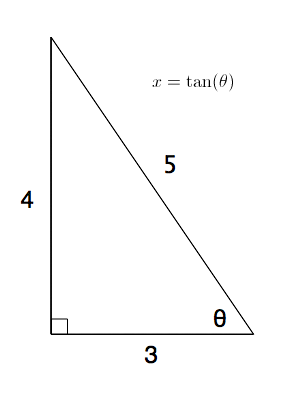#### Question 2 2. What is the following radian measure in degrees?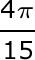#### Question 3 3. Convert the following measurement in radian to degrees.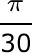#### Question 4 4. What's the value of 2sin(θ)cos(θ) given that the equation below is true?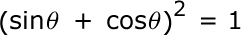#### Question 5 5. What is x?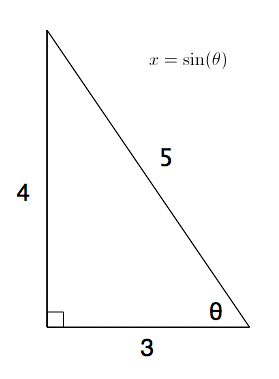### Page 2

#### Question 6 6. What is x?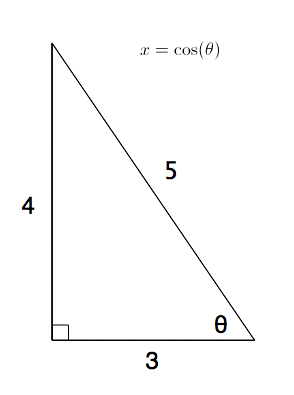#### Question 10 10. What is the value of cos(θ) given that the equation below is true?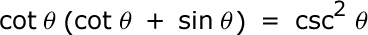### Page 3

#### Question 11 11. What's the value of sec2 (θ) given that the equation below is true?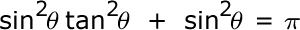#### Question 13 13. Perform the following calculation. Report the answer in radians.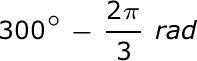#### Question 15 15. The lines a and b are parallel lines of length 5 and 2. The lines d and e are parallel to one another, and both are perpendicular to a and b. What is the length of c?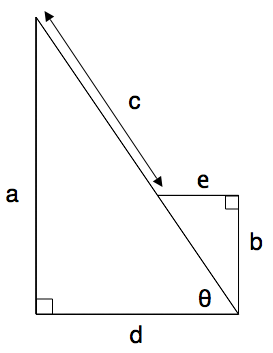### Page 4

#### Question 17 17. Simplify the following expression.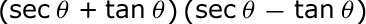#### Question 18 18. Simplify the following expression. Report the answer in degrees.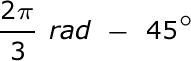#### Question 19 19. What is x?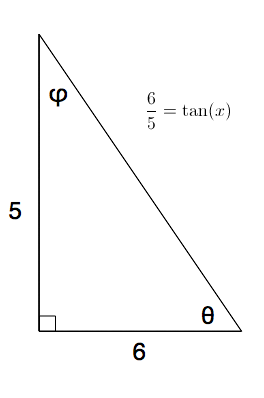#### Question 20 20. Simplify the following expression.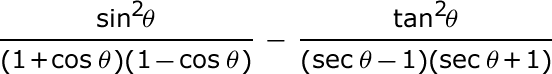#### Common Core HS Functions - Trigonometric Functions Chapter Exam Instructions

Choose your answers to the questions and click 'Next' to see the next set of questions. You can skip questions if you would like and come back to them later with the yellow "Go To First Skipped Question" button. When you have completed the practice exam, a green submit button will appear. Click it to see your results. Good luck!

Support